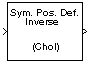# Cholesky Inverse

Compute inverse of Hermitian positive definite matrix using Cholesky factorization

## Library

Math Functions / Matrices and Linear Algebra / Matrix Inverses

`dspinverses`

•## Description

The Cholesky Inverse block computes the inverse of the Hermitian positive definite input matrix S by performing Cholesky factorization.

`${S}^{-1}={\left(L{L}^{\ast }\right)}^{-1}$`

L is a lower triangular square matrix with positive diagonal elements and L* is the Hermitian (complex conjugate) transpose of L. Only the diagonal and upper triangle of the input matrix are used, and any imaginary component of the diagonal entries is disregarded. Cholesky factorization requires half the computation of Gaussian elimination (LU decomposition), and is always stable.

### Response to Nonpositive Definite Input

The algorithm requires that the input be Hermitian positive definite. When the input is not positive definite, the block reacts with the behavior specified by the Non-positive definite input parameter. The following options are available:

• `Ignore` — Proceed with the computation and do not issue an alert. The output is not a valid inverse.

• `Warning` — Display a warning message in the MATLAB® Command Window, and continue the simulation. The output is not a valid inverse.

• `Error` — Display an error dialog box and terminate the simulation.

Note

The Non-positive definite input parameter is a diagnostic parameter. Like all diagnostic parameters on the Configuration Parameters dialog box, it is set to `Ignore` in the code generated for this block by Simulink® Coder™ code generation software.

## Parameters

Non-positive definite input

Response to nonpositive definite matrix inputs: `Ignore`, `Warning`, or `Error`. See Response to Nonpositive Definite Input.

## References

Golub, G. H., and C. F. Van Loan. Matrix Computations. 3rd ed. Baltimore, MD: Johns Hopkins University Press, 1996.

## Supported Data Types

• Double-precision floating point

• Single-precision floating point

 Cholesky Factorization DSP System Toolbox Cholesky Solver DSP System Toolbox LDL Inverse DSP System Toolbox LU Inverse DSP System Toolbox Pseudoinverse DSP System Toolbox `inv` MATLAB

See Matrix Inverses for related information.

## Extended Capabilities

Introduced before R2006a

## SupportGet trial now